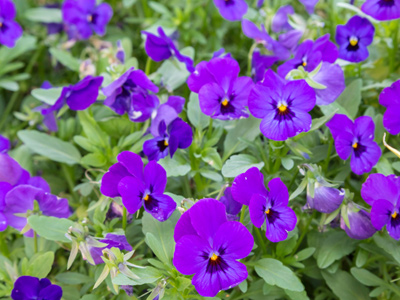Hannah gives her pot plant 30 ml of water each day. How much water will the plant require in a year?

# Level 3-4 Shapes - Measuring Capacity

As part of your KS3 Maths work on shapes you will be used to measuring heights, widths, areas, angles, circumferences and perimeters. These are all relevant to 2D shapes like squares or circles. 3D shapes have one other aspect - volume or capacity.

We can work out the capacities of 3D shapes by using certain formulae. Litres and millilitres are two examples of measurements of capacity or volume. These are metric measurements and so very easy to work with - provided that you are careful with noughts and decimal places. Occasionally you may come across imperial units of capacity like gallons or pints. But don't worry - this quiz sticks to metric measures.

To make you familiar with millilitres and litres and how they relate to one another you can play this quiz. Take your time and read each question carefully before submitting your answers. Good luck!.

1.
A level teaspoon is approximately 5 ml. How many level teaspoons in 1.5 litres?
20
30
300
3,000
1.5l = 1,500ml so to find the answer divide 1,500 by 5
2.
A barrel of oil contains 159 litres. How many barrels will be required for 795 litres?
3
4
5
6
795 ÷ 159 = 5
3.
Hannah gives her pot plant 30 ml of water each day. How much water will the plant require in a year (365 days)?
3,650 ml
7,300 ml
7.3 litres
10.95 litres
365 days x 30 ml = 10,950 ml of water required. To convert millilitres to litres divide by 1,000
4.
You need to know the capacity of a watering can that is full of water and all you have to help you is a half litre jug. How do you find the approximate capacity of the watering can?
Count how many times you can fill the jug from the can
Estimate the weight of the can and multiply by two
Time how long it takes to fill the jug from the can
Guess
The number of times the jug is filled multiplied by 500 ml will give you the capacity of the watering can
5.
Jake has three cups of tea every day but he never drinks an entire cup. On average he throws away 25 millilitres each cup. Approximately how much tea does he waste in a calendar month consisting of 30 days?
75 ml
750 ml
1.25 litres
2.25 litres
3 (cups) x 30 (days) x 25 (ml waste) = 2,250 millilitres or 2.25 litres
6.
There are 1,000 litres of sand in a sandpit. If a bucket holds 11 litres, approximately how many buckets of sand are in the sandpit?
91
99
100
101
1,000 ÷ 11 = 90.90909090909091. We can round this number up to 91
7.
What are the abbreviations for litre and millilitre?
l and ml
le and me
lt and mt
lit and mil
1,000 ml (millilitres) = 1 l (litre)
8.
Sophie drinks 500 millilitres of orange juice every day. How long will it take her to drink 20 litres?
20 days
40 days
60 days
80 days
500ml = 0.5l so, to find the answer divide 20 by 0.5
9.
A litre of water weighs a kilogram. How many litres will there be in 5 tonnes of water?
500
1,000
5,000
50,000
1,000 kilograms - 1 tonne
10.
A container that will hold a litre of milk is three quarters full. How many millilitres of milk are in the container?
250
500
750
1,250
There are 1,000ml in 1 litre. Three quarters of 1,000 is 750